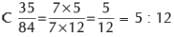# Olympiad Test: Ratio And Proportion - 2

## 20 Questions MCQ Test Math Olympiad for Class 5 | Olympiad Test: Ratio And Proportion - 2

Description
Attempt Olympiad Test: Ratio And Proportion - 2 | 20 questions in 40 minutes | Mock test for Class 5 preparation | Free important questions MCQ to study Math Olympiad for Class 5 for Class 5 Exam | Download free PDF with solutions
QUESTION: 1

Solution:
QUESTION: 2

### The ratio 35 : 84 in simplest form is:

Solution:QUESTION: 3

### In a class there are 20 boys and 15 girls. The ratio of boys to girls is:

Solution:
QUESTION: 4

Two numbers are in the ratio 7: 9. If the sum of the numbers is 112, then the larger number is:

Solution:
QUESTION: 5

The ratio of 1.5 m to 10 cm is:

Solution:
QUESTION: 6

The ratio of 1 hour to 300 seconds is:

Solution:

1 hr = 60 x 60 = 3600

1 hr : 300 secs = 3600/300 = 12:1

QUESTION: 7

In 4 : 7 : : 16 : 28, 7 and 16 are called

Solution:
QUESTION: 8

The first, second and fourth terms of a proportion are 16, 24 and 54 respectively. Then the third term is:

Solution:
QUESTION: 9

If 12, 21, 72, 126 are in proportion, then:

Solution:
QUESTION: 10

If x, y and z are in proportion, then:

Solution:

Three quantities are said to be in continued proportion; if the ratio between the first and the second is equal to the ratio between the second and the third.

Thus,x,y  and z are in continued proportion

QUESTION: 11

7 : 12 is equivalent to:

Solution:
QUESTION: 12

The length and breadth of a rectangle are in the ratio 3 : 1. If the breadth is 7cm, then the length of the rectangle is:

Solution:
QUESTION: 13

The value of m, if 3, 18, m, 42 are in proportion is:

Solution:
QUESTION: 14

Length and width of a field are in the ratio 5: 3. If the width of the field is 42 m then its length is:

Solution:
QUESTION: 15

If a:b:c=3:4:7, then the ratio (a+b+c):c is equal to

Solution:

2 : 1
(a+b+c) = 3 + 4 + 7 = 14 and c = 7
Then, (a+b+c) : c= 2 : 1.

QUESTION: 16

The number of students in 3 classes is in the ratio 2:3:4. If 12 students are increased in each class this ratio changes to 8:11:14. The total number of students in the three classes in the beginning was

Solution:

162
Let number of students in the classes be
2x, 3x and 4x respectively; then
total students= 2x+3x+4x=9x.
According to question, (2x+12)/ (3x+12) = 8/11
⇒ 24x+96=22x+132
2x= 132 – 96
⇒ x= 36/2= 18
Hence, original number of students,
= 9x= 9 × 18=162.

QUESTION: 17

If 2/3 of A=75% of B=0.6 of C, then A:B:C is

Solution:

9 : 8 : 10
According to question, Or, (2A/3)=(75B/100)=(C × 6/10); A × 2/3= B × 3/4;
⇒ A/B= 9/8;
And,
B × 3/4= C × 3/5;
⇒ B/C= 8 : 10;
Thus, A : B : C= 9 : 8 : 10.

QUESTION: 18

If A and B are in the ratio 3:4, and B and C in the ratio 12:13, then A and C will be in the ratio

Solution:

9 : 13
(A/B) × (B/C) = (3/4) × (12/13);
Or, A/B= 36/39= 9 : 13.

QUESTION: 19

If A : B = 2 : 3 and B : C = 4 : 5 then A : B : C is

Solution:

A : B : C
= A : B
B : C
= 2 : 3
4 : 5
= 8 : 12 : 15

QUESTION: 20

In a school having roll strength 286, the ratio of boys and girls is 8 : 5. If 22 more girls get admitted into the school, the ratio of boys and girls becomes

Solution:

Boys : girls = 8 : 5; (let be boys = 8x; girl= 5x)
Total strength= 286; 8x + 5x= 286; 13x = 286;
Or, x= 286/13=22;
Boys= 176 and girls= 110;
22 more girls get admitted then number of girls become,
(5x + 22)= 110+22= 132;
Now, ratio of boys and girls
= 176 : 132 = 4 : 3Use Code STAYHOME200 and get INR 200 additional OFF Use Coupon Code